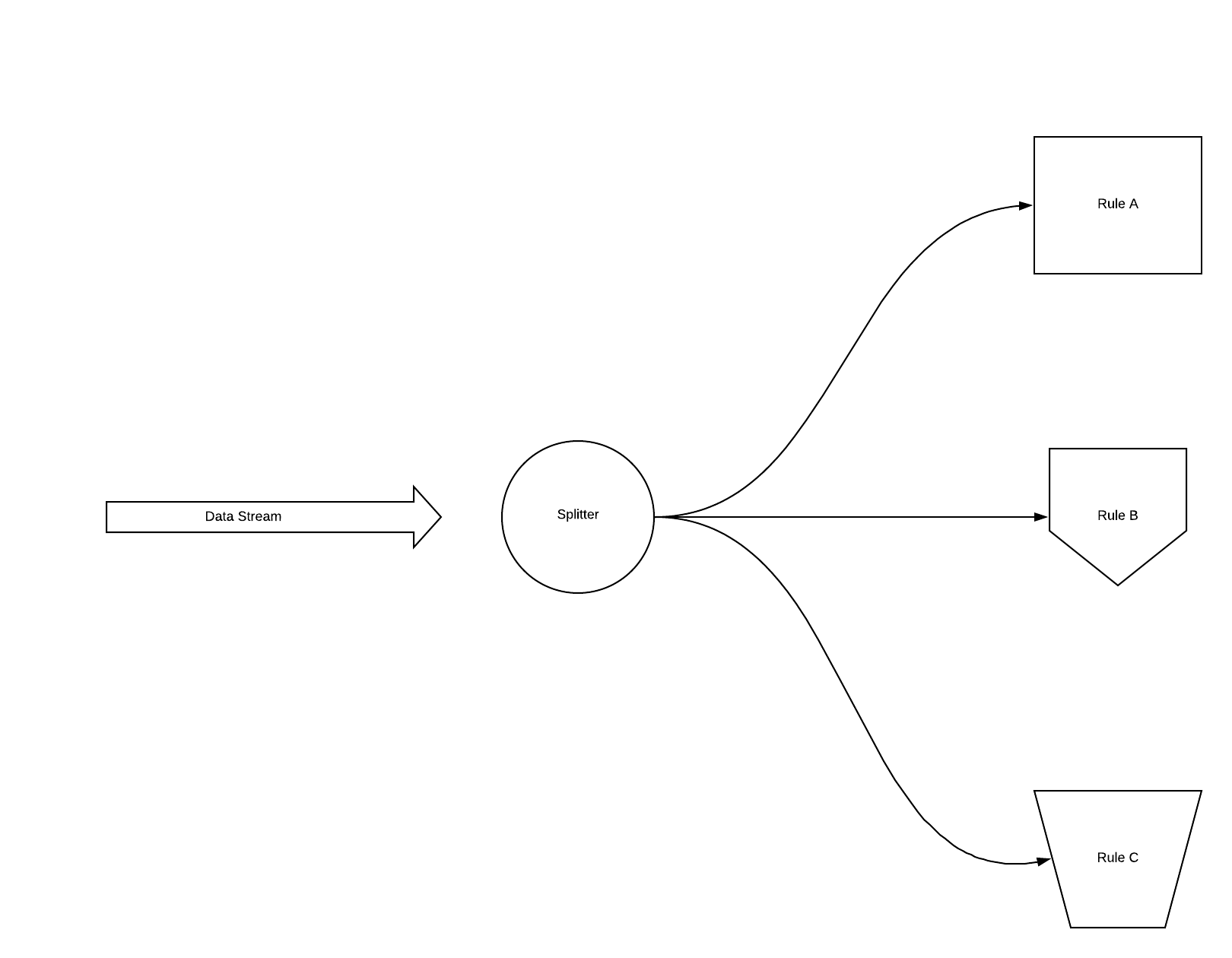A couple of weeks ago, I got assigned to a story where I need to create a rule engine. The rule engine has a feature that the user able to create a configuration to assign specific rules to obtain a percentage of the incoming payload. For example, the user can assign rule A to receive 20 percent of the payload, rule B to receive 40 percent of the payload, and rule C to receive 40 percent of the payload.

You have seen it in a lot of A/B testing framework. You configure the system to receive 40 percent of the user traffic to render text A while 60 percent of the user traffic to render text B.

You also have seen it happens in the “smart” load balancing assignment algorithm where you can automatically configure 20 percent to server A, 30 percent to server B, and 50 percent to server C.

How do these A/B testing frameworks and load balancer assign feature work under the hood without knowing the amount of data that is coming?

In this article, I would like to share about how I tackled this problem, and I hope my answer can also benefit others that also encounter the same problem.

At first, I searched for all related articles regarding stream algorithms. However, those stream algorithm ended up having much statistical analysis and very complex to implement.

The incoming payload doesn’t need to be precise. It needs to receive an estimated percentage number on each assignment. Therefore, after research, I concluded two solutions.

## Roll the dice

Roll the dice solution is based on the random generator to choose which section to assign the element from the stream.

Imagine if you have a 70-30 chance of assigning the incoming element to A or B.

Roll the dice when you receive the incoming element. If the dice number is between 1 to 4.2, assign the value to A. If the dice number is between 4.3 to 6, assign the value to B.

One of the advantages of this approach is that it is easy to implement.

Run a random generator when receiving the element. If the result is between 0 to 60, assign to A. If the result is between 70 to 99, assigned to B.

import scala.util.Random

var A = 0 // 20%
var B = 0 // 30%
var C = 0 // 50%

(1 to 100000).foreach{ _ =>
val random = new Random().nextInt(100)
if(random < 20) {
A += 1
} else if(random >= 20 && random <50) {
B += 1
} else {
C += 1
}
}

println(s"${A}${B} \${C}")


If you run the function above over and over again, you can see that the roughly estimated amount be 20 - 30 - 50.

However, if the application did not have a large amount of data to consume, using a random generator to split elements might cause disproportionate on the data consumption for A and B.

If there are only 100 incoming data, using roll the dice might cause 20 percent of the data to A and 80 percent of the data to B.

Since the assignment is estimated, we can be sure from the law of large numbers that the algorithm closes to accurate.

## Buffer Solution

Another solution is to use a cache to put the incoming element until it hits a fixed number. When the cache hits the fixed number, assign the data set into either A and B based on the percentage.

I call this buffer solution - it is like buffering where it waits until the number of incoming bytes and assign all of them at once before flushing all the bytes to the output stream.

Imagine the same above example where you need to assign 70 percent of the traffic to A and 30 percent to B.

Using the buffer solution, you create a cache that store 1000 incoming data stream. Once the cache reaches 1000 incoming data, assign 70 percent of that incoming data to A and 30 percent to B. Then, flush out by letting cache data go through. Retrieve the next 1000 data in the cache.

This solution solves the problem of roll the dice solution, where the data may not be distributed evenly. However, the buffer solution is much harder to implement and maintain. You need to introduce a caching ability in the system and maintain the state later on. The performance of the system may also suffer since there is an additional IO that involves in the system.

These two solutions are the solution that I proposed after countless research on the internet and reading some white paper. However, this solution can be optimized by creating a more precise probability as it shifts away from the desired state.

If you have any other suggested solutions or how to solve this problem, please share your solution below.

## Source

javascript - Randomly split up elements from a stream of data without knowing the total number of elements - Stack Overflow

## Subscribe

* indicates required

#### Related Posts

##### Create a Throttling System for Any Application with no more than 100 lines of Code

With the help of functional programming and FS2

##### How to Write Data to a File with FS2

A More Performant Function